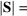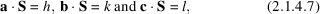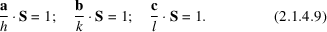International
Tables for
Crystallography
Volume F
Crystallography of biological macromolecules
Edited by E. Arnold, D. M. Himmel and M. G. Rossmann

International Tables for Crystallography (2012). Vol. F, ch. 2.1, pp. 55-56   | 1 | 2 |

## Section 2.1.4.5. Scattering by a crystal

J. Drentha*

aLaboratory of Biophysical Chemistry, University of Groningen, Nijenborgh 4, 9747 AG Groningen, The Netherlands
Correspondence e-mail: j.drenth@chem.rug.nl

#### 2.1.4.5. Scattering by a crystal

| top | pdf |

A unit cell contains a large number of electrons, especially in the case of biological macromolecules. The waves scattered by these electrons interfere with each other, thereby reducing the effective number of electrons in the scattered wave. The exception is scattering in the forward direction, where the beams from all electrons are in phase and add to each other. The effective number of scattering electrons is called the structure factor F because it depends on the structure, i.e. the distribution of the atoms in the unit cell. It also depends on the scattering direction. If small electron-density changes due to chemical bonding are neglected, the structure factor can be regarded as the sum of the scattering by the atoms in the unit cell, taking into consideration their positions and the corresponding phase differences between the scattered waves. For n atoms in the unit cellwhere S is a vector perpendicular to the plane reflecting the incident beam at an angle θ; the length of S is given by[equation (2.1.4.3)in Section 2.1.4.2].

The origin of the system is chosen at the origin of the selected unit cell. Atom j is at positionwith respect to the origin. Another unit cell has its origin atand, where t, u and v are whole numbers, and a, b and c are the basis vectors of the unit cell. With respect to the first origin, its scattering isThe wave scattered by a crystal is the sum of the waves scattered by all unit cells. Assuming that the crystal has a very large number of unit cells, the amplitude of the wave scattered by the crystal isFor an infinitely large crystal, the three summations over the exponential functions are delta functions. They have the property that they are zero unlesswhere h, k and l are whole numbers, either positive, negative or zero. These are the Laue conditions. If they are fulfilled, all unit cells scatter in phase and the amplitude of the wave scattered by the crystal is proportional to the amplitude of the structure factor F. Its intensity is proportional to.

S vectors satisfying equation (2.1.4.7)are denoted by S(hkl) or S(h), and the corresponding structure factors asor F(h).

Bragg's law for scattering by a crystal is better known than the Laue conditions:where d is the distance between reflecting lattice planes, θ is the reflecting or glancing angle and λ is the wavelength (Fig. 2.1.4.9).It can easily be shown that the Laue conditions and Bragg's law are equivalent by combining equation (2.1.4.7)with the following information:

• (1) Vector S is perpendicular to a reflecting plane (Section 2.1.4.2).Figure 2.1.4.9 | top | pdf |X-ray diffraction by a crystal is, in Bragg's conception, reflection by lattice planes. The beams reflected by successive planes have a path difference of, where d is the lattice-plane distance and θ is the reflecting angle. Positive interference occurs if,,etc., where λ is the X-ray wavelength. Reproduced with permission from Drenth (1999). Copyright (1999) Springer-Verlag.
• (2) The Laue conditions for scattering [equation (2.1.4.7)]can be written as• (3) Lattice planes always divide the unit-cell vectors a, b and c into a number of equal parts (Section 2.1.1). If the lattice planes divide the a vector of the unit cell into h equal parts, the first index for this set of planes is h. The second index, k, is related to the division of b and the third index, l, to the division of c.

From equation (2.1.4.9)it follows that vector S(hkl) is perpendicular to a plane determined by the points a/h, b/k and c/l, and according to conditions (3)this is a lattice plane. Therefore, scattering by a crystal can indeed be regarded as reflection by lattice planes. The projection of a/h, b/k and c/l on vector S(hkl) is(Laue condition), but it is also equal to the spacingbetween the lattice planes (see Fig. 2.1.1.3),and, therefore,. Combining this with equation (2.1.4.3)yields Bragg's law,[equation (2.1.4.8)].